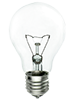Use the sliders to calculate your estimated kWh usage and cost. Do you know your bulb Wattage or general Watt total use ? Use the chart below to estimate Watts based on your current type and number of bulbs.

###### LED###### 5-9W

Example; 10 x 60W incandescent bulbs = 600W – set the first slider to 600W, etc.

Default; 1 x 60W incandescent bulb

Customer rate of \$0.129 per kWh, rate varies per region and utility provider.

The difference between watts (W), kilowatts (kW), and kilowatt-hours (kWh).

## Watts

Unit of electrical power equal to 1 joule per second. Light bulbs are rated in watts to indicate how much energy they consume.

A 60W incandescent bulb typically gives off around 650-800 lumens. Watts are a measure of how much energy the light bulb will use as it produces light. Lumens are the measure of how much light the bulb produces.

###### LED## What is a kilowatt (kW)?

Kilowatts are a measure of how much energy something consumes.

Watts (W) to kilowatts (kW): 1kW is equal to 1,000W.

To convert W to kW, divide the total wattage by 1,000.

Ten 100W light bulbs is equal 1kW of energy usage.

10 bulbs x 100W = 1,000W

1,000W / 1,000 = 1kW

Your energy usage depends on how long you keep everything on.

## What is a kilowatt-hour (kWh)?

kWh is the unit of measurement that quantifies how much energy is used over a period of time, calculated by multiplying the kW of energy consumption by the total number of hours the lighting has been operated.

Ten 100W light bulbs.

10 bulbs X 100W = 1,000W or 1kW of lighting

10 hours of daily use X 30 days in the month = 300 hours of use

1kW X 300 hours of use = 300kWh of energy consumption

A significant portion of your electricity bill is based on your energy consumption in kilowatt-hours.